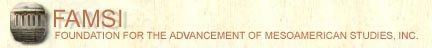John Montgomery
Dictionary of Maya Hieroglyphs

﻿

I

To hear the sounds and learn more, click on the text below the glyph.

Search the dictionary for words in English or Maya.

A | B' | CH | CH' | E | H | I | J | K | K' | L | M | N | O | P | S | T | T' | TZ | TZ' | U | W | X | Y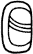-il (-il)
(T24)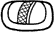-il (-il)
(T24)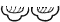-il (-il)
(T360)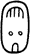-il (-il)
(T82)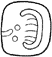-il (-il)
(T82)-il (-il)
(T83)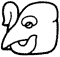-il (-il)
(Tnn)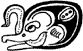-il (-il)
(Tnn)i (i)
(T237v)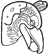i (i)
(T237v)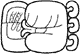I-IL-ji (ilij)
(T679.618v.136)i-ka-tzi (ikatz)
(T679:25:124)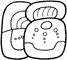i-ka-tzi (ikatz)
(T679.25:507)i-ki-tzi (ikitz)
(T679.102:507)i-tz'a-ta (itz'at)
(T58:103)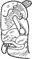i-tz'i-{n} (itz'in)
(T237v)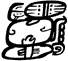i-tz'i-{n} wi-WINIK-ki (itz'in winik)
(T679.248:521:103.117)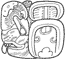i-tz'i-{n} WINIK (itz'in winik)
(T237v.248:521)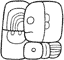i-u-ti (i-ut)
(T679.513:59)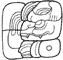i-u-ti (i-ut)
(T679.738:59)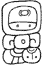i-yu-wa-la (i-yuwal)
(T679:61:177)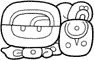i-yu-wa-la (i-yuwal)
(T679:62.130:534)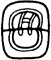i/I (i)
(T679)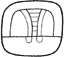i/I (i)
(T679)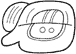i/I (i)
(T87?.512)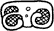ICH'AK' (ich'ak')
(T5)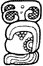ICH'AK' TUN-ni (ich'ak' tun)
(T5:528:116)ICH'AK'-ki (ich'ak')
(Tnn:102)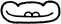IK' (Ik')
(T23)IK' (Ik')
(T503)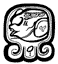IK' (Ik')
(T503hv)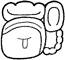IK' K'U (Ik' K'u)
(T23:503.36?)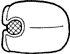IL (il)
(T618v)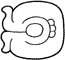IL-ja (ilaj)
(T618v)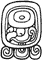IMIX (Imix)
(T501hv)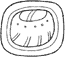IMIX (Imix)
(T501)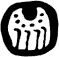IMIX (Imix)
(T501)IN (in)
(T116)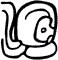IN CH'EN (in ch'en)
(T116.598v)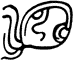IN KAB' (in kab')
(T116.526)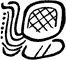IN pa-ta (in pat)
(T116.586:103?)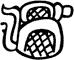IN tu-pa (in tup)
(T116.92:586)IN-b'u-ku (in b'uk)
(T116.21:528)ITZ' (itz')
(T152)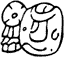itz'-am-{na} (Itz'amná)
(T152.nn)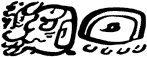ITZ'AM ye-ji (Itz'am Yej)
(T152.nn 512.136)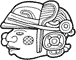ITZ'AT (itz'at)
(Tnn)ITZ'AT (itz'at)
(Tnn)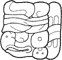ITZ'AT-ta (itz'at)
(Tnn.102)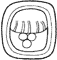IX (Ix/ix)
(T524)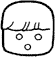IX (Ix/ix)
(T524)IX TZ'AM(?) (ix tz'am?)
(T524:150)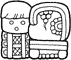IX TZ'AM(?) ku-aj (Ix Tz'am? Ku-aj)
(T524:150.528:12)IX/IXIK (ix/ixik)
(T1000b)IXIK-ki (ixik)
(T1000b:102)
Index | Next Page
Return to top of page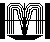Order John Montgomery’s Book